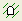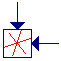The Rocscience International Conference 2021 Proceedings are now available. Read Now

# Ubiquitous Joints

The Ubiquitous Joints option allows you to plot strength factor contours which account for jointing in the rock mass. The term "ubiquitous" means that the joints may occur at any point in the rock mass, they do not have a fixed location.

IMPORTANT NOTE: ubiquitous joint modeling in RS2 is a post-analysis feature, using the stresses calculated from the finite element analysis – the ubiquitous joint strength factor calculations have no effect on the stresses or displacements, which have already been calculated.

To plot Ubiquitous Joint strength factor contours:

1. The Ubiquitous Joint properties (strength and orientation) are defined by selecting the Define Ubiquitous Jointsoption from the toolbar or the Data menu. The properties of up to 3 sets of Ubiquitous Joints can be defined. See below for details.
2. To plot Ubiquitous Joint contours:
• Select the Display button in the Define Ubiquitous Joints dialog, OR
• At any time, you can select Ubiquitous Jointsfrom the drop-list of data types in the Interpret toolbar.
3. The strength factor contours will account for the Ubiquitous Joint properties currently defined in the Define Ubiquitous Joints dialog.

4. You can change the ubiquitous joint properties at any time. In the Define Ubiquitous Joints dialog, edit the properties you wish to change, select Display, and the strength factor plot will be updated to reflect the new joint properties.

The following properties may be entered in the Define Ubiquitous Joints dialog.

## Number of Joint Sets

You may select One, Two, or Three, to enter properties for up to three different joint sets.

## Slip Criterion

You may select either Mohr-Coulomb or Barton-Bandis strength parameters for the ubiquitous joints.

Strength Factor

You may choose to plot the strength factor considering the ubiquitous joints only, or considering both the ubiquitous joint strength and the rock mass strength. This works as follows:

• At each node of the mesh, the strength factor is calculated for EACH joint set using the Mohr-Coulomb or Barton-Bandis slip criterion properties you have entered. See below for further details about the strength factor calculation.
• If you have defined more than one joint set (i.e. 2 or 3 joint sets), then the MINIMUM of these represents the ubiquitous joint strength factor at each node.
• The Joints Only option will ignore the rock mass strength factor, and contour the strength factor for the ubiquitous joints ONLY.
• The Joints and Rock option will contour the LESSER of the ubiquitous joint strength factor OR rock mass strength factor at each node.

To close the dialog and plot the contours, select the Display button.

Ubiquitous Joint Strength Factor Calculation

At any point within the rock mass, the normal and shear stress can be computed on a plane at any orientation using solid mechanics. So given a joint orientation, the normal and shear stress can be computed on the plane. Given the normal stress on a plane, the shear strength can also be computed on the same plane. The factor of safety (strength factor) is simply the shear strength divided by the shear stress. You can do this for any number of joint orientations but we only allow for three in RS2. The ubiquitous joints do not influence the stress/displacement field, they are simply a post-processing tool to determine whether the stresses generated from the finite element analysis are sufficient to cause slip on a plane at a user defined orientation.

## Tensile Stress and Strength Factor

If the normal stress on a ubiquitous joint plane is tensile, then the shear strength (and the strength factor) for the joint is automatically set to zero.

For the Mohr-Coulomb model this implies that the tensile cutoff is zero. For the Barton-Bandis model the shear strength is zero by definition for tensile normal stress. In either case, the ubiquitous joint shear strength (and strength factor) will be zero if the normal stress on the plane is tensile.

## Display of Ubiquitous Joint Planes

When viewing ubiquitous joint strength factor plots, if the Stress Block is displayed, the ubiquitous joint slip planes will be indicated in the Stress Block, as shown in the figure below. This is useful as a quick check of the angles you have entered for the Inclination of each joint set. If the angle(s) appear wrong, check the inclination(s) you have entered in the Define Ubiquitous Joints dialog.

Stress Block icon with 3 ubiquitous joint planes (inclinations = -50 , 20 and 70 degrees)